Are you more of a visual learner? Check out our online video lectures and start your physics course now for free!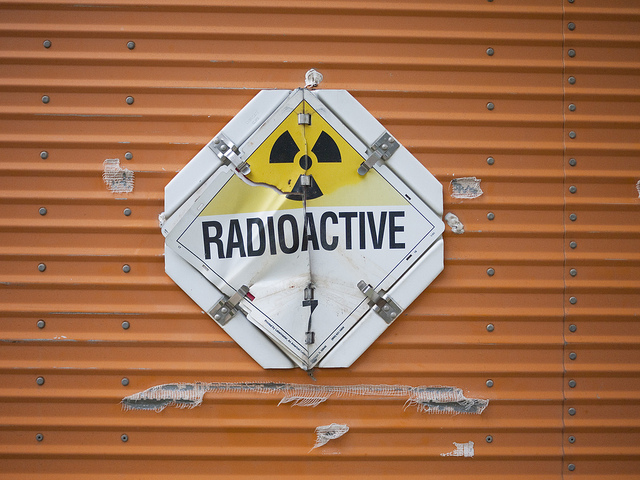In 1896, Henri Becquerel discovered a hitherto unknown, non-continuous ray that could penetrate many materials—including black paper—leading to fogging of photographic plates. Two years later, Pierre and Marie Curie, conducting experiments on uranium ore and uranium pitchblende, discovered 2 radioactive elements known as polonium and radium.

Radiation that carries sufficient energy to free negatively-charged electrons from their atoms or molecules is known as ionizing radiation leaving behind positively-charged atoms. Unstable atomic nuclei are transformed spontaneously into other atoms, which results in ionizing radiation. This phenomenon is known as radioactivity.

Naturally occurring radioactive materials include the elements radium and potassium. Natural radioactivity occurs during the stabilization of a radionuclide resulting in the emission of alpha, beta, and gamma rays.

Above a specific dosage, all radioactive materials, especially those that emit ionizing radiation, become a health hazard.

The transformation in which the nucleus of an unstable atom loses energy via radiation emission is known as radioactive decay. The timing of radioactive decay of an atom cannot be predicted and is not a subject of external influence.

### Activity

The nuclear decay rate A (activity) is the quotient resulting after dividing the number of decays N by the amount of time t:

A = dN / dt

Activity is measured in units of becquerel [Bq]. An obsolete unit is the Curie, which was named after its discoverer Marie Curie in 1896. One Curie is equivalent to 3.7 * 1010 decays per second.

If a mass is also factored into the equation, it is known as specific radioactivity—which is the decay by a specific concentration of radionuclide atoms. In this case, its mass may refer to that of the:

• the chemical element (isotopes included) or compound.
• the entire sample.

The actual activity is an important characteristic of radioactive material and is easy to calculate. If N is the number of the decaying nuclei in the substance and λ is the decay rate, the activity of the radioactive substance can be calculated as:

A = λ * N

### Decay Constant

Every element, or more specifically, every atom, has its own specific decay constant, which is the probability of a specific type of nuclear decay independent of time and place.

N.B. Every radionuclide is associated with a different decay constant!

### Half-Life

The time required for the number of radioactive atoms to reduce to half its original value is known as the half-life.

Half-life (T1/2) = ln2 / λ

In this time frame, the number N of the radioactive nuclei of an element is reduced to half of its initial value N0.

N = N0 * (1/2)1/T

Every radioactive isotope has its own individual half-life.

### Wave Types

• α-rays: Alpha rays (α-rays) are made of 2 protons and 2neutrons bound together into a particle identical to a positively-charged helium nucleus. Due to their positive charge, they can be deflected by electrical and magnetic fields. Alpha rays have a velocity of around 107 m/s.
• β-rays: Beta rays (β-rays) are high-energy electron rays similar to those in cathode rays. They are made of electrons with a velocity ranging between 108 m/s and 0.99 c0. Due to their negative charge, they are deflected by electrical and magnetic fields. The rays are deflected in the direction opposite to alpha particles.
• γ-rays: Gamma rays (γ-rays) are high-frequency electromagnetic waves with wavelengths of about 10-12 m and frequencies around 1020 Hz. Gamma rays are not deflected by electrical or magnetic fields.

When atoms are artificially transformed, isotopes can be left behind which emit the 4th kind of radioactive wave:

• β+-rays: The β+-rays are positive electrons carrying the same mass but opposite charge as electrons, and are called positrons.

The range of radioactive waves depends on the particle type and on the radioactive substance itself. Alpha radiation, for example, has a low range of only 4–8 cm.

Absorption of radioactive waves: Alpha rays can be stopped by a sheet of paper, while 1-mm-thick aluminum plates are necessary to stop beta waves. Thick lead walls are required to absorb gamma rays.

Elements produce radioactive waves during their transformation into other elements. During this process, they produce large amounts of thermal energy. During elemental conversion, an atom will only ever emit one type of ray, either alpha or beta. They are never emitted together at the same time. Gamma rays, however, are typically emitted along with alpha or beta radiation.

If different radioactive materials are mixed together, like in a sample of non-pure radium, all 3 types of rays are present. The radium atom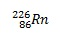can lose a helium nucleus (i.e., alpha radiation) and convert into the noble gas radon: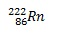Radon decays to radium A by emitting alpha radiation. Radium A emits further alpha rays to convert into radium B. Radium B emits beta and gamma rays until it is transformed into radium C. This element transformation continues leading to radium C1, radium D, radium E, and so on until the element becomes stable, i.e., no longer radioactive.

In contrast to historical names such as Radium A, B, and C, the names of modern elements are used in the following reaction equations.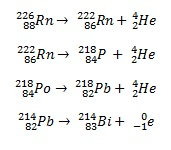Atomic stability: The ratio of the number of neutrons N to the number of protons Z increases proportionate to the atomic mass number. Atoms are stabilized once a specific ratio of neutrons to protons is reached.

The prerequisites for a stable atomic nucleus are as follows:

N/Z ~ 1 + 0,015 A2/3 with A < 250

A … the mass number (N + Z)

N … the number of neutrons in the nucleus

Z … the number of protons in the nucleus

α-decay

Alpha decay (α-decay) is a type of radioactive decay generating an atom with an atomic number that is reduced by 2.

Example: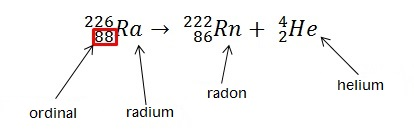α-decay occurs only in nuclei that carry high mass numbers.

β-decay

Beta-decay (β-decay) entails the transformation of a proton into a neutron, or vice versa, inside the atomic nucleus. The atomic nucleus is converted into a nucleus with an atomic number increased by one.

Example: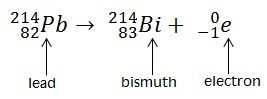β-decay draws an atom closer to the optimal ratio of protons and neutrons. During this transformation, the nucleus emits a detectable beta particle, which is an electron or positron.

β-decay, in the case of a relative excess of neutrons, emits an electron when a neutron is transformed into a proton. In the case of β+-decay, atoms possess an excess of protons. Here, a proton is converted into a neutron while emitting a positron.

γ-decay

During gamma decay (γ-decay), the charge of an atom remains unchanged. Therefore, the atomic number remains the same. Gamma decay usually occurs after other forms of decay such as α or β-decay. The atomic nuclei relax from an excited state into lower-energy states.

Atomic decay represents statistical behavior. Due to a large number of atoms contained in radioactive materials, it is possible to formulate the laws of radioactive decay. The number of decayed unstable nuclides in a given period of time t is directly proportional to the number N0 of existing radioactive nuclides initially, the period itself, and the decay constant of the nuclide.

ΔN = λ * N0 * Δt

### Dosimetry

The effect of radiation on the body or an object exposed to the radiation is determined by the energy transferred to that body. This effect applies not only to radiation emitted by radioactive substances but also to all ionizing radiations such as X-rays or neutron rays.

The absorbed dose D is calculated by the ratio of energy E received by that body to the mass m of the body. The physical quantity of absorbed radiation is measured by the unit gray (Gy).

D = E / m

The absorbed dosage is the ratio of the dose D to the exposure time.

D’ = D / t

The ionizing effect is one of the principal characteristics of radiation. The number of ions present in the air is a measure of the radiation intensity. The ion dose or exposure is calculated by measuring the ratio of ions in the air Q and the mass m of the irradiated air:

Ion dose J = Q / m

Ion dose J generated by a specific gamma-ray with the activity of 1 becquerel from a distance of 1 m per second is indicated by the specific gamma constant. The ion dose is expressed by the corresponding energy dose, given that the required amount of energy to ionize a molecule is known for all substances.

It is important to determine the impact of ionizing radiation on living tissue when dealing with radiation protection issues.

## X-Rays

Wilhelm Conrad Röntgen discovered X-rays while working with experimental discharge tubes. He found that invisible rays can penetrate matter that is otherwise impenetrable to ‘normal’ light. He called this kind of radiation X-rays, also referred to as Röntgen rays in honor of his name.

Electromagnetic waves with photon energies in the range of 100 eV and several MeV are referred to as X-rays.

X-ray tube construction: An X-ray tube consists of a cathode (usually tungsten) inside a vacuum, which is connected to a high voltage filament. Electrons exit the cathode via thermionic emission and are collected by an anode on the opposite side. A high voltage between the cathode and the anode accelerates the electrons from the cathode to the anode. A fluorescent screen is placed before the X-ray tube.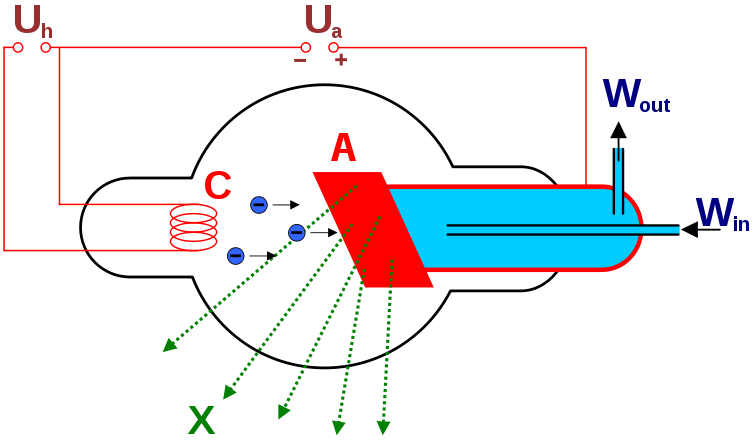Image: Coolidge side-window tube (scheme) C: filament/cathode (-) A: anode (+) Win and Wout: water inlet and outlet of the cooling device. By von Hmilch, License: Public Domain

Electrons exit the cathode and collide with the tungsten anode, which emits photons that receive some of the kinetic energy. Invisible rays illumine the fluorescent screen via glass transition. The entire system is located in a vacuum in order to function.

The amount of photon energy depends on the amount of energy transferred to the photon, which varies greatly each time. If all the kinetic energy is transferred to a single photon, it is referred to as maximum photon energy:

Emax = Ekin = e * UB

The amount of available energy depends on the degree of accelerating voltage UB, i.e., the higher the voltage, the faster is the movement of electrons before they hit the anode, and thus the energy of the photons is increased. If the heating voltage is higher, additional electrons separate from the cathode.

Learn. Apply. Retain.
Your path to achieve medical excellence.
Study for medical school and boards with Lecturio.(Votes: 5, average: 5.00)Loading...